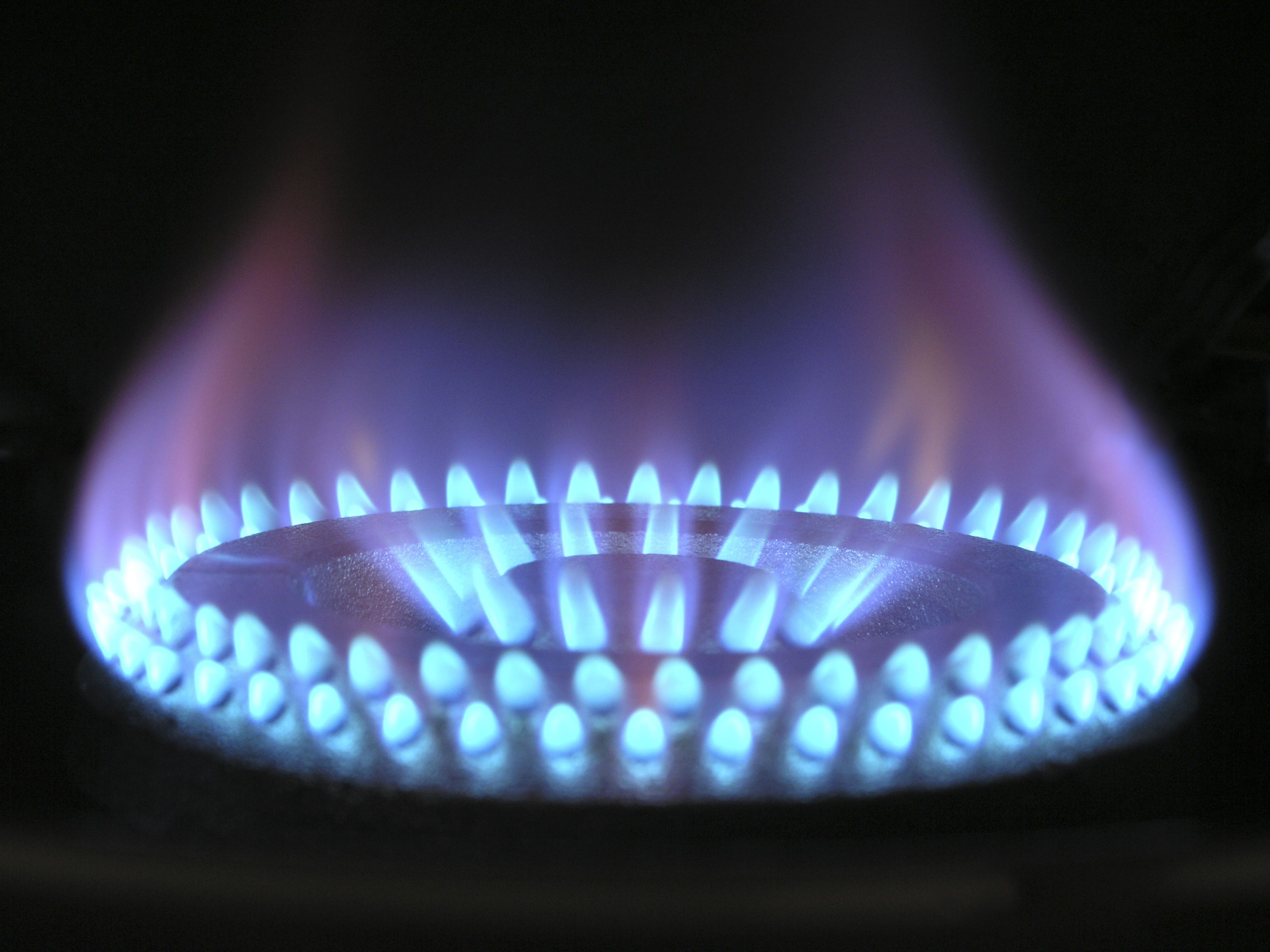# How to Calculate the Size of an Anaerobic Digester TankHi! This is a bonus track on Anaerobic Digestion. What we'll do is we'll calculate the size of a fixed-dome reactor for the example of a boarding school. Okay. This is the situation.

A boarding school with 250 students and about 50 staff in a tropical climate. We have around 0.2 kg of wet waste, biowaste, per capita a day. The TS is around 20% and the volatile solids are estimated around 80% of the TS. The gas shall be used in the canteen of the boarding school.We want to build a fixed dome reactor below ground. The picture is here to illustrate what this should look like, and we know that the construction and operational skills are available on site. So, what should we calculate? First, let's look at the things related to feedstock. We have 300 people x 0.

2 kg per day of biowaste per capita. That amounts to 60 kg per day of wet waste. And we have a TS of 20% of this wet waste. We'll shred this waste into small pieces and mix it with one part waste to two parts water so that we can create a slurry that could feed nicely into the digester.

So that means 1 x 60 of wet waste, plus 2 x 60 of water, equals 180 litres, assuming one kilogram equals one litre.

Next, let's look at retention time. For a digester in a tropical climate, we can assume a good retention time would be around 30 days. So that means, 180 litres per day x 30 days equals 5,400 litres, which is equal to 5.4 cubic meters. Now let's look at feedstock quality.

We have 60 kg of wet waste, we have a TS of 20%, so that equals 12 kg of dry matter. And we have 80% volatile solids, so times this 12 kg of dry matter equals 9.6 kg of volatile solids per day per 180 litres. But now what we want to have is the amount per cubic meter. So, the calculation that we need to do is 9.

6 / 180 x 1,000 equals to 53.3 kg of volatile solids per cubic meter. Now let us look at the organic loading rate. The organic loading rate is the flow rate times the concentration divided by the reactor volume. The units are, for flow rate, cubic meters per day; for concentration — kilograms of volatile solids per cubic meter and for the reactor volume, cubic meters.

Now let's plug in the numbers. So that is 0.18 cubic meters per day, that the 180 litres, 53.3 kg volatile solids per cubic meter. That is just what we calculated previously.

And 5.4 cubic meters – that's the volume that we calculated based on the retention time. That equals 1.78 kg of volatile solids per cubic meter. And if you remember the slides that we showed previously, when talking about the organic loading rate for unstirred reactors, it is ideal to have an organic loading rate below 2.

So we have achieved this in our calculation. Now let's look at the amount of gas that we expect. From the literature, we can expect a biogas yield for vegetable waste of around 0.67 cubic meters per kilogram of volatile solids. So we have 1.

78 kg pf volatile solids per cubic meter, which we just calculated before, times the 0.67 cubic meters per kilogram of volatile solids, times the reactor volume of 5.4 cubic meters. That equals to 6.4 cubic meters per day.

That means we can expect 6.4 cubic meters of biogas production per day. Also from literature, we know that a biogas stove uses about 0.4 cubic meters per hour. We have this 6.4 cubic meters per day, so we know that if we use one stove, we actually operate it for 16 hours per day, or, if we have four stoves, we can operate them each for four hours per day. So that means, we can actually cook quite a lot of food in the canteen of the boarding school. Now let's look at the total volume of the unit. In this specific design, we estimate that we need about 75% of the total volume for the slurry and 25% as gas holder storage. So, we have 5.

4 cubic meters of slurry, plus an additional 1.8 cubic meters for the gas holder, equals the total volume that we need for the whole reactor, which is 7.2 cubic meters.

So let's sum up what we calculated. We calculated the amount of feedstock, 180 litres.

We calculated the volume of the digester based on the retention time of 30 days, which is 5.4 cubic meters. We looked at total volatile solids per cubic meter, 53.3, and to check on our organic loading rate, we calculated the organic loading rate which is 1.78, which is nicely below 2, so it fits nicely with our system.

And we calculated the amount of gas, 6.4 cubic meters per day, looked at how much a biogas stove consumes and calculated how long we actually operate biogas stoves.

Finally, we also calculated the total volume of the unit also considering the volume that we need for the gas holder, and it amounts total to 7.2 cubic meters. So that was the calculation for a fixed-dome reactor.

I hope you can use it in your case in your country.